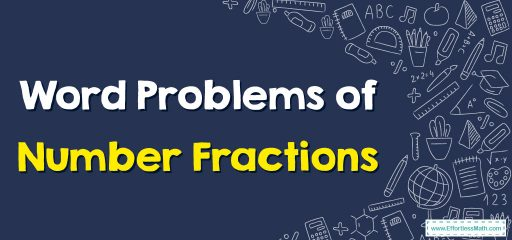# Word Problems: FractionsWord problem is a or two sentences that require students to apply their math skills and whatever they have learned to a real-life scenario.
Some of the word problems of number fractions are:

• Addition and subtraction of fractions
• Dividing fractions
• Multiplication of fractions
• Simplification of fractions

### Word Problems of Number Fractions – Examples 1

Kevin has 9 chocolates. He wants to share them with 5 friends. How much will each get?

Solutions:

Step 1: Divide 9 chocolates by 5+his=6:
Step 2: Then, simplify the product if it is possible.

$$9÷6=\frac{9}{1}÷\frac{6}{1}=\frac{9}{1}×\frac{1}{6}=\frac{9×1}{1×6}=\frac{9}{6}$$

$$\frac{9}{6}=\frac{3}{2}$$

### Word Problems of Number Fractions – Examples 2

Alice needs to order grilled chicken for 15 students. Each student should get $$\frac{1}{5}$$ of a grilled chicken. How many grilled chickens should Alice order?

Solutions:

Step 1: Multiply 15 grilled chickens by $$\frac{1}{5}$$.
Step 2: Then, simplify the product if it is possible.

$$15×\frac{1}{5}=\frac{15}{1}×\frac{1}{5}=\frac{15}{5}\frac{3}{1}=3$$

### What people say about "Word Problems: Fractions - Effortless Math: We Help Students Learn to LOVE Mathematics"?

No one replied yet.

X
30% OFF

Limited time only!

Save Over 30%

SAVE $5 It was$16.99 now it is \$11.99